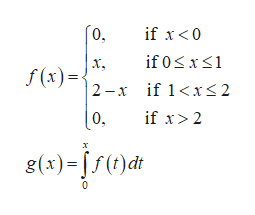# Let f(x) = 0 if x < 0 x if 0 ≤ x ≤ 1 2 − x if 1 < x ≤ 2 0 if x > 2 and g(x) = x f(t) dt 0 . andg(x) = xf(t) dt 0.(a) Find an expression for g(x) similar to the one for f(x).

Question
382 views

Let f(x) = 0 if x < 0 x if 0 ≤ x ≤ 1 2 − x if 1 < x ≤ 2 0 if x > 2 and g(x) = x f(t) dt 0 . and

g(x) =
 x f(t) dt 0
.
(a) Find an expression for g(x) similar to the one for f(x).
check_circle

Step 1

The given function arehelp_outlineImage Transcriptionclose[о. if 0 xs1 if x<0 х, f(x) |2 -х if 12 х 8(3)-Ј/0jd fullscreen
Step 2

Suppose

Step 3

Suppose...

### Want to see the full answer?

See Solution

#### Want to see this answer and more?

Solutions are written by subject experts who are available 24/7. Questions are typically answered within 1 hour.*

See Solution
*Response times may vary by subject and question.
Tagged in

### Integration